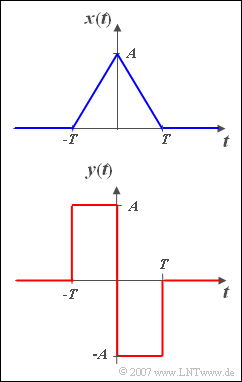# Exercise 3.5: Differentiation of a Triangular PulseTriangular signal and
differentiated triangular signal

We are looking for the spectrum  $Y(f)$  of the signal

$$y\left( t \right) = \left\{ \begin{array}{c} A \\ - A \\ 0 \\ \end{array} \right.\quad \begin{array}{*{20}c} {{\rm{f or}}} \\ {{\rm{for}}} \\ \\ \end{array}\;\begin{array}{*{20}c} { - T \le t < 0,} \\ {0 < t \le T,} \\ {{\rm{else}}{\rm{.}}} \\\end{array}$$

Let  $A = 1\,{\rm V}$  and  $T = 0.5\,{\rm ms}$ apply.

The Fourier transform of the triangular pulse  $x(t)$ sketched above is assumed to be known, namely

$$X( f ) = A \cdot T \cdot {\mathop{\rm si}\nolimits} ^2 ( {{\rm{\pi }}fT} ),$$

where  $\text{si}(x) = \text{sin}(x)/x$.

A comparison of the two signals shows that the following relationship exists between the functions  $x(t)$  and  $y(t)$ :

$$y(t) = T \cdot \frac{{{\rm d}x(t)}}{{{\rm d}t}}.$$

Hints:

### Questions

1

Calculate the spectral function  $Y(f)$  at the output. What is its magnitude at the frequencies  $f = 0$  and  $f = 1 \ \rm kHz$?

 $|Y(f=0)| \hspace{0.2cm} = \$  $\text{mV/Hz}$ $|Y(f=1 \ \text{kHz})| \ = \$  $\text{mV/Hz}$

2

Which statements are true regarding the spectrum  $Y(f)$ ?

 The zeros of  $X(f)$  also remain in  $Y(f)$ . For large  $f$–values,  $Y(f)$  satisfies the same bound as  $X(f)$. For large  $f$–values,  $|X(f)|$  is smaller than the magnitude spectrum of a rectangular pulse of duration  $T$.

3

Calculate  $Y(f)$  starting from the rectangular pulse by applying the displacement theorem. Which statement is true here?

 The differentiation theorem leads to the result more quickly. The shifting theorem leads to the result more quickly.

### Solution

#### Solution

(1)  The differentiation theorem reads generally:

$$\frac{{{\rm d}x( t )}}{{{\rm d}t}}\circ\!\!-\!\!\!-\!\!\!-\!\!\bullet\,{\rm{j}} 2{\rm{\pi }}f \cdot X( f ).$$
• Applied to the present example, one obtains:
$$Y( f ) = T \cdot {\rm{j}}\cdot 2{\rm{\pi }}f \cdot A \cdot T \cdot \frac{{\sin ^2 ( {{\rm{\pi }}fT} )}}{{( {{\rm{\pi }}fT} )^2 }} = {\rm{j}} \cdot 2 \cdot A\cdot T \cdot \frac{{\sin ^2 ( {{\rm{\pi }}fT} )}}{{{\rm{\pi }}fT}}.$$
• This function is purely imaginary. At the frequency  $f = 0$  the imaginary part also disappears. This can be formally proven, for example, by applying l'Hospital's rule  ⇒   $Y( f = 0 ) \;\underline{= 0}$.
• However, the result also follows from the fact that the spectral value at  $f = 0$  is equal to the integral over the time function  $y(t)$ .
• At the normalised frequency  $f \cdot T = 0.5$  $($i.e for  $f = 1\,\text{ kHz})$  the sine function is equal to  $1$  and we obtain  $|Y(f = 1 \,\text{kHz})| = 4/\pi \cdot A \cdot T$, i.e. approximately  $|Y(f=1 \ \text{kHz})| \ \underline{=0.636 \,\text{ mV/Hz}}$  (positive imaginary).

(2)  The correct solutions are 1 and 3:

• The zeros of  $X(f)$  remain and there is another zero at the frequency  $f = 0$.
• The upper bound is called the asymptotic curve
$$\left| {Y_{\max }( f )} \right| = \frac{2A}{{{\rm{\pi }} \cdot |f|}} \ge \left| {Y( f )} \right|.$$
• For the frequencies at which the sine function delivers the values  $\pm 1$  ,  $|Y_{\text{max}}(f)|$  and  $|Y(f)|$  are identical.
• For the rectangular pulse of same amplitude  $A$  the corresponding bound is  $A/(\pi \cdot |f|)$.
• In contrast, the spectrum  $X(f)$  of the triangular pulse falls asymptotically faster:
$$\left| {X_{\max }( f )} \right| = \frac{A}{{{\rm{\pi }}^{\rm{2}} f^2 T}} \ge \left| {X( f )} \right|.$$
• This is due to the fact that  $x(t)$  has no discontinuity points.

(3)  Starting from a symmetrical rectangular pulse  $r(t)$  with amplitude  $A$  and duration  $T$  the signal  $y(t)$  can also be represented as follows:

$$y(t) = r( {t + T/2} ) - r( {t - T/2} ).$$
• By applying the shifting theorem twice, one obtains:
$$Y( f ) = R( f ) \cdot {\rm{e}}^{{\rm{j\pi }}fT} - R( f ) \cdot {\rm{e}}^{ - {\rm{j\pi }}fT} .$$
• Using the relation  $\text{e}^{\text{j}x} – \text{e}^{–\text{j}x} = 2\text{j} \cdot \text{sin}(x)$  it is also possible to write for this:
$$Y( f ) = 2{\rm{j}} \cdot A \cdot T \cdot {\mathop{\rm si}\nolimits}( {{\rm{\pi }}fT} ) \cdot \sin ( {{\rm{\pi }}fT} ).$$
• Consequently, the result is the same as in subtask  (1).
• Which way leads faster to the result, everyone must decide for himself. The author thinks that the first way is somewhat more favourable.
• Subjectively, we decide in favour of solution suggestion 1.